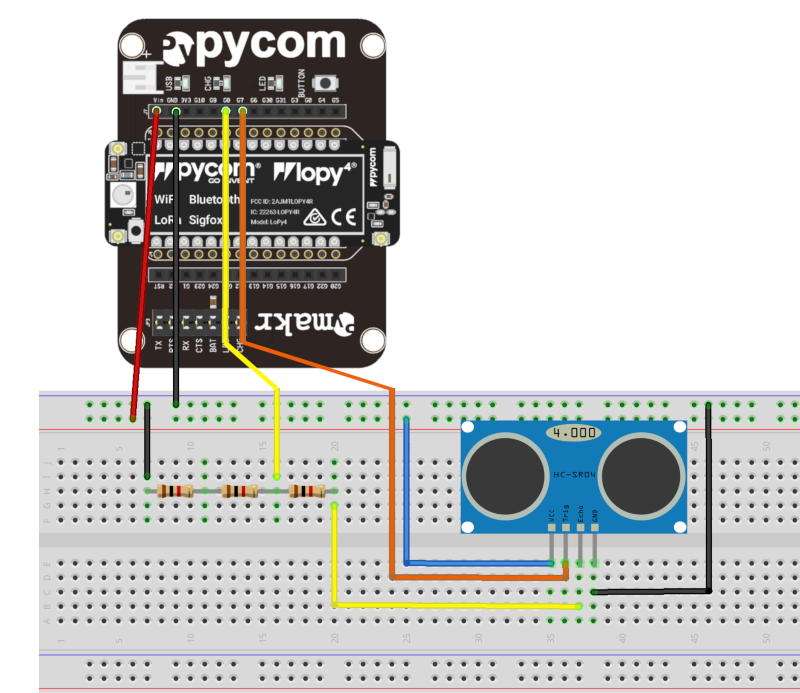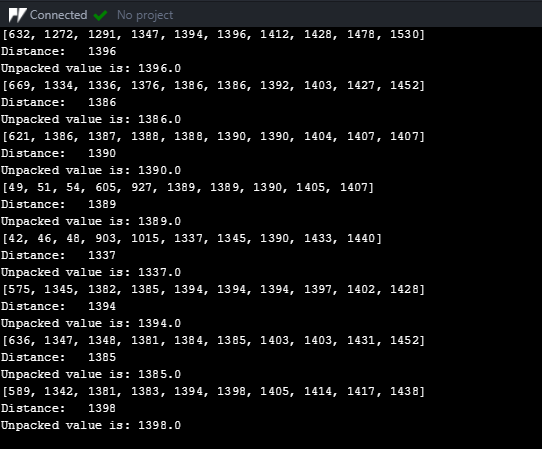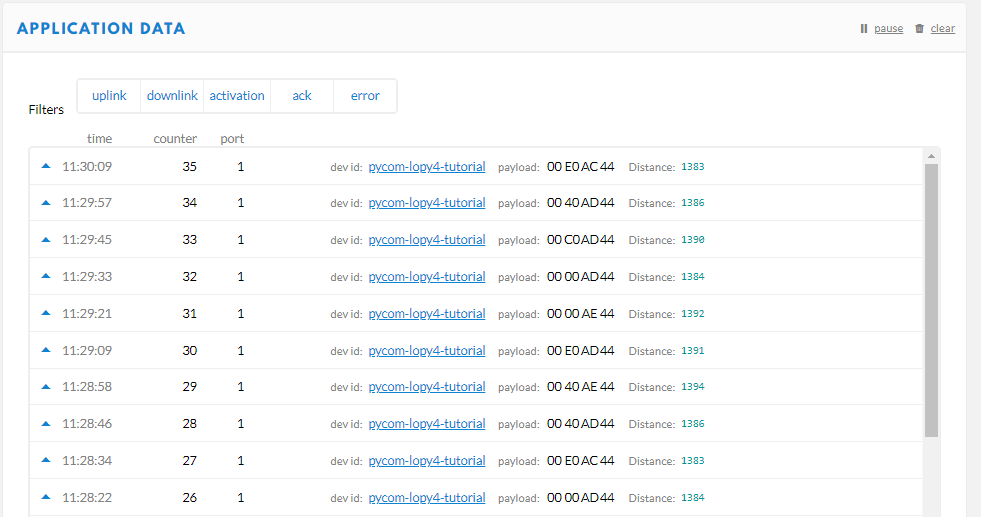empowering creative people

# Ultrasonic Sensing with Pycom

One of the most common sensors in any makers toolbox is the HC-SR04 Ultrasonic Rangefinder. They are an easy to use solution for detecting the proximity of objects up to 6m away! They work best in the 1-2 meter range though. In this tutorial, we will be using the Pycom Lopy4 to take readings from an HC-SR04 Rangefinder.

## How it works

The ultrasonic rangefinder works by sending out a 10us pulse of sound at 40,000hz. The sound bounces off an object and travels back to the sensor. The length of the received echo is proportional to the distance that the sound travelled. We can measure the length of the return pulse and calculate the distance. Using the formula:

Time = Distance / Speed

The time is measured in microseconds with the sensor, and we know the speed of sound is 340m/s or .034cm/us. We then need to divide the result by 2 because the distance will be how far the pulse travelled there and back. The final formula is:

Distance = (Time(us) * .034) / 2

## The Circuit

The HC-SR04 is a 5V device, and Pycom boards are all 3.3V. We can power the sensor using the VIN pin on the expansion board ONLY if we are connected to USB. If we are running on batteries then we won't have the 5V needed and will need to power the sensor using a regulator or a separate 5V source. The 3.3V logic from the Pycom boards works to trigger the sensor.

We also need to step down the echo voltage from 5V to 3.3V. We've done this by using a voltage divider. We had 1k resistors handy but you could use a 1k and a 2.2k resistor instead.## The Code

Despite its apparent complexity, the HC-SR04 is an analog sensor. This means that the measurements made using this sensor need to be controlled on the microcontroller. In this example, we will be using the Pycom Lopy4 to take readings and then upload the results to The Things Network. You could use this code with any Pycom board, just leave out the LoRa parts!

### Taking Measurements

This code is the bare bones needed to take distance measurements using the HC-SR04 Ultrasonic Rangefinder. We will be using the utime library because there is a function in it that will be unaffected by the clock rolling over. We initialize the echo and trigger pin that we are connecting the sensor to. In this example, we are using the Expansion board, if you are connecting directly to your Pycom board then use the relevant pin on the board itself. Just refer to the Pinout Diagram to find its number.

`import utimeimport pycomimport machinefrom machine import Pin# initialise Ultrasonic Sensor pinsecho = Pin(Pin.exp_board.G7, mode=Pin.IN) # Lopy4 specific: Pin('P20', mode=Pin.IN)trigger = Pin(Pin.exp_board.G8, mode=Pin.OUT) # Lopy4 specific Pin('P21', mode=Pin.IN)trigger(0)# Ultrasonic distance measurmentdef distance_measure():    # trigger pulse LOW for 2us (just in case)    trigger(0)    utime.sleep_us(2)    # trigger HIGH for a 10us pulse    trigger(1)    utime.sleep_us(10)    trigger(0)    # wait for the rising edge of the echo then start timer    while echo() == 0:        pass    start = utime.ticks_us()    # wait for end of echo pulse then stop timer    while echo() == 1:        pass    finish = utime.ticks_us()    # pause for 20ms to prevent overlapping echos    utime.sleep_ms(20)    # calculate distance by using time difference between start and stop    # speed of sound 340m/s or .034cm/us. Time * .034cm/us = Distance sound travelled there and back    # divide by two for distance to object detected.    distance = ((utime.ticks_diff(start, finish)) * .034)/2    return distancewhile True:    print(distance_measure())`

### Eliminating False Readings

`    # to reduce errors we take ten readings and use the mediandef distance_median():    # initialise the list    distance_samples = []    # take 10 samples and append them into the list    for count in range(10):        distance_samples.append(int(distance_measure()))    # sort the list    distance_samples = sorted(distance_samples)    # take the center list row value (median average)    distance_median = distance_samples[int(len(distance_samples)/2)]    # apply the function to scale to volts    print(distance_samples)    return int(distance_median)`

### The Big Picture

Now that we have the basics down, we will add in everything we need to get the ultrasonic sensor sending data to The Things Network via LoRaWAN!

```from network import LoRa
import socket
import utime
import binascii
import pycom
import ustruct
import machine
from machine import Pin

# initialise Ultrasonic Sensor pins
echo = Pin(Pin.exp_board.G7, mode=Pin.IN) # Lopy4 specific: Pin('P20', mode=Pin.IN)
trigger = Pin(Pin.exp_board.G8, mode=Pin.OUT) # Lopy4 specific Pin('P21', mode=Pin.IN)
trigger(0)

# Ultrasonic distance measurment
def distance_measure():
# trigger pulse LOW for 2us (just in case)
trigger(0)
utime.sleep_us(2)
# trigger HIGH for a 10us pulse
trigger(1)
utime.sleep_us(10)
trigger(0)

# wait for the rising edge of the echo then start timer
while echo() == 0:
pass
start = utime.ticks_us()

# wait for end of echo pulse then stop timer
while echo() == 1:
pass
finish = utime.ticks_us()

# pause for 20ms to prevent overlapping echos
utime.sleep_ms(20)

# calculate distance by using time difference between start and stop
# speed of sound 340m/s or .034cm/us. Time * .034cm/us = Distance sound travelled there and back
# divide by two for distance to object detected.
distance = ((utime.ticks_diff(start, finish)) * .034)/2

return distance

# to reduce errors we take ten readings and use the mediandef distance_median():    # initialise the list    distance_samples = []    # take 10 samples and append them into the list    for count in range(10):        distance_samples.append(int(distance_measure()))    # sort the list    distance_samples = sorted(distance_samples)    # take the center list row value (median average)    distance_median = distance_samples[int(len(distance_samples)/2)]    # apply the function to scale to volts    print(distance_samples)    return int(distance_median)

# disable LED heartbeat (so we can control the LED)
pycom.heartbeat(False)
# set LED to red
pycom.rgbled(0x7f0000)

# lora config
lora = LoRa(mode=LoRa.LORAWAN, region=LoRa.AS923)
# access info
app_eui = binascii.unhexlify('70B3D57ED0012FC2')
app_key = binascii.unhexlify('6C3B6BD79C939C85AF19786EA4120057')

# attempt join - continues attempts background
lora.join(activation=LoRa.OTAA, auth=(app_eui, app_key), timeout=0)

# wait for a connection
print('Waiting for LoRaWAN network connection...')
while not lora.has_joined():
utime.sleep(1)
# if no connection in a few seconds, then reboot
if utime.time() > 15:
print("possible timeout")
machine.reset()
pass

# we're online, set LED to green and notify via print
pycom.rgbled(0x004600)
print('Network joined!')

# setup the socket
s = socket.socket(socket.AF_LORA, socket.SOCK_RAW)
s.setsockopt(socket.SOL_LORA, socket.SO_DR, 5)
s.setblocking(False)
s.bind(1)

count = 0
# limit to 200 packets; just in case power is left on
while count < 200:

# take distance measurment, turn the light blue when measuring
pycom.rgbled(0x00007d)
utime.sleep(1)
distance = distance_median()
pycom.rgbled(0x004600)

print("Distance:  ", distance)
# encode the packet, so that it's in BYTES (TTN friendly)
# could be extended like this struct.pack('f', distance) + struct.pack('c',"example text")
# 'h' packs it into a short, 'f' packs it into a float, must be decoded in TTN
packet = ustruct.pack('h', distance)

# send the prepared packet via LoRa
s.send(packet)

# example of unpacking a payload - unpack returns a sequence of
#immutable objects (a list) and in this case the first object is the only object
print ("Unpacked value is:", ustruct.unpack('h',packet))

# check for a downlink payload, up to 64 bytes
rx_pkt = s.recv(64)

# check if a downlink was received
if len(rx_pkt) > 0:
print("Downlink data on port 200:", rx_pkt)
pycom.rgbled(0xffa500)
input("Downlink recieved, press Enter to continue")
pycom.rgbled(0x004600)

count += 1
utime.sleep(10)
```

Now we have data coming into the serial monitor!## The Things Network

When we run this program we will take 10 distance readings in a burst, and return the median value. That value is then encoded and sent to The Things Network as a payload. To be able to read it on TTN we will need to decode it. In the "Payload Formats" on our application page within TTN, we will use the following javascript decoder to unpack our data from bytes:

```function Decoder(bytes, port) {
// Decode an uplink message from a buffer
// (array) of bytes to an object of fields.
var decoded = {};

// Decode bytes to int
var testShort = (bytes << 8) | bytes;

// Decode int
decoded.Distance = testShort;

return decoded;
}```

Now that our decoder is entered we should be getting human-readable data flowing in!## Going Further

In this tutorial, we demonstrated the basics of using the HC-SR04, but we didn't talk much about connecting to TTN and using LoRaWAN with the Pycom Lopy4. If you want to learn more about LoRaWAN head over to our The Things Network Tutorials. There is even a Tutorial on Encoding and Decoding Payloads for The Things Network. If you want to learn more about using Pycom Devices, check out our Pycom Tutorials!

Have a question? Ask the Author of this guide today!